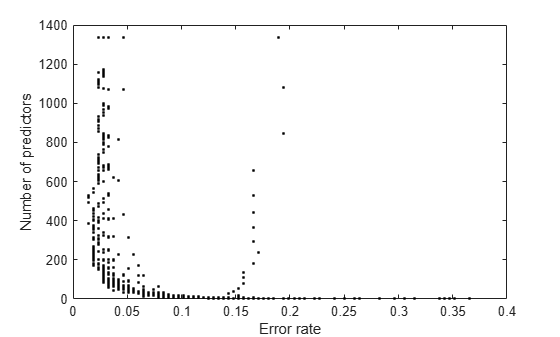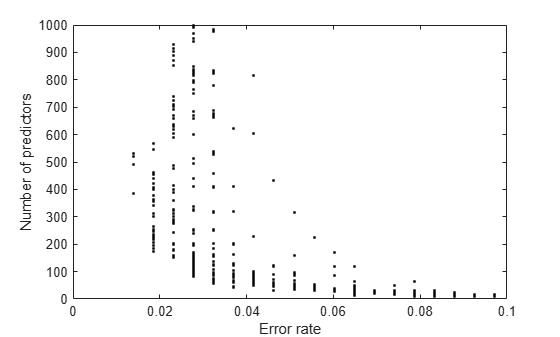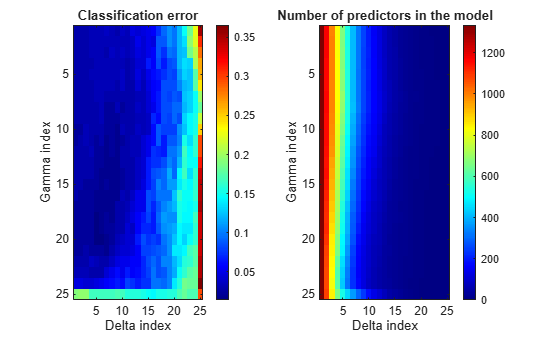# Regularize Discriminant Analysis Classifier

This example shows how to make a more robust and simpler model by trying to remove predictors without hurting the predictive power of the model. This is especially important when you have many predictors in your data. Linear discriminant analysis uses the two regularization parameters, Gamma and Delta, to identify and remove redundant predictors. The `cvshrink` method helps identify appropriate settings for these parameters.

### Load data and create a classifier.

Create a linear discriminant analysis classifier for the `ovariancancer` data. Set the `SaveMemory` and `FillCoeffs` name-value pair arguments to keep the resulting model reasonably small. For computational ease, this example uses a random subset of about one third of the predictors to train the classifier.

```load ovariancancer rng(1); % For reproducibility numPred = size(obs,2); obs = obs(:,randsample(numPred,ceil(numPred/3))); Mdl = fitcdiscr(obs,grp,'SaveMemory','on','FillCoeffs','off');```

### Cross validate the classifier.

Use 25 levels of `Gamma` and 25 levels of `Delta` to search for good parameters. This search is time consuming. Set `Verbose` to `1` to view the progress.

```[err,gamma,delta,numpred] = cvshrink(Mdl,... 'NumGamma',24,'NumDelta',24,'Verbose',1);```
```Done building cross-validated model. Processing Gamma step 1 out of 25. Processing Gamma step 2 out of 25. Processing Gamma step 3 out of 25. Processing Gamma step 4 out of 25. Processing Gamma step 5 out of 25. Processing Gamma step 6 out of 25. Processing Gamma step 7 out of 25. Processing Gamma step 8 out of 25. Processing Gamma step 9 out of 25. Processing Gamma step 10 out of 25. Processing Gamma step 11 out of 25. Processing Gamma step 12 out of 25. Processing Gamma step 13 out of 25. Processing Gamma step 14 out of 25. Processing Gamma step 15 out of 25. Processing Gamma step 16 out of 25. Processing Gamma step 17 out of 25. Processing Gamma step 18 out of 25. Processing Gamma step 19 out of 25. Processing Gamma step 20 out of 25. Processing Gamma step 21 out of 25. Processing Gamma step 22 out of 25. Processing Gamma step 23 out of 25. Processing Gamma step 24 out of 25. Processing Gamma step 25 out of 25. ```

### Examine the quality of the regularized classifiers.

Plot the number of predictors against the error.

```plot(err,numpred,'k.') xlabel('Error rate') ylabel('Number of predictors')```Examine the lower-left part of the plot more closely.

`axis([0 .1 0 1000])`There is a clear tradeoff between lower number of predictors and lower error.

### Choose an optimal tradeoff between model size and accuracy.

Multiple pairs of `Gamma` and `Delta` values produce about the same minimal error. Display the indices of these pairs and their values.

First, find the minimal error value.

`minerr = min(min(err))`
```minerr = 0.0139 ```

Find the subscripts of `err` producing minimal error.

`[p,q] = find(err < minerr + 1e-4);`

Convert from subscripts to linear indices.

`idx = sub2ind(size(delta),p,q);`

Display the `Gamma` and `Delta` values.

`[gamma(p) delta(idx)]`
```ans = 4×2 0.7202 0.1145 0.7602 0.1131 0.8001 0.1128 0.8001 0.1410 ```

These points have as few as 29% of the total predictors with nonzero coefficients in the model.

`numpred(idx)/ceil(numPred/3)*100`
```ans = 4×1 39.8051 38.9805 36.8066 28.7856 ```

To further lower the number of predictors, you must accept larger error rates. For example, to choose the `Gamma` and `Delta` that give the lowest error rate with 200 or fewer predictors.

```low200 = min(min(err(numpred <= 200))); lownum = min(min(numpred(err == low200))); [low200 lownum]```
```ans = 1×2 0.0185 173.0000 ```

You need 173 predictors to achieve an error rate of 0.0185, and this is the lowest error rate among those that have 200 predictors or fewer.

Display the `Gamma` and `Delta` that achieve this error/number of predictors.

```[r,s] = find((err == low200) & (numpred == lownum)); [gamma(r); delta(r,s)]```
```ans = 2×1 0.6403 0.2399 ```

### Set the regularization parameters.

To set the classifier with these values of `Gamma` and `Delta`, use dot notation.

```Mdl.Gamma = gamma(r); Mdl.Delta = delta(r,s);```

### Heatmap plot

To compare the `cvshrink` calculation to that in Guo, Hastie, and Tibshirani , plot heatmaps of error and number of predictors against `Gamma` and the index of the `Delta` parameter. (The `Delta` parameter range depends on the value of the `Gamma` parameter. So to get a rectangular plot, use the `Delta` index, not the parameter itself.)

```% Create the Delta index matrix indx = repmat(1:size(delta,2),size(delta,1),1); figure subplot(1,2,1) imagesc(err) colorbar colormap('jet') title('Classification error') xlabel('Delta index') ylabel('Gamma index') subplot(1,2,2) imagesc(numpred) colorbar title('Number of predictors in the model') xlabel('Delta index') ylabel('Gamma index')```You see the best classification error when `Delta` is small, but fewest predictors when `Delta` is large.

 Guo, Y., T. Hastie, and R. Tibshirani. "Regularized Discriminant Analysis and Its Application in Microarray." Biostatistics, Vol. 8, No. 1, pp. 86–100, 2007.